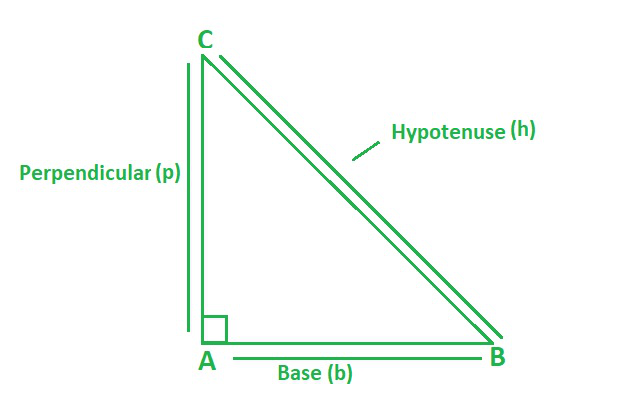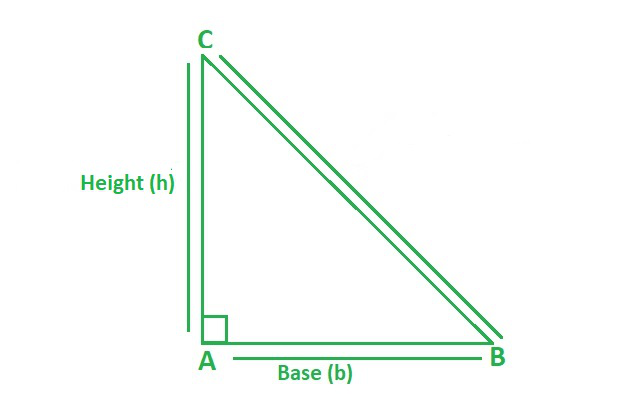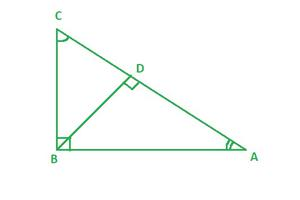Open in App
Not now

# Right Triangle Formula

• Last Updated : 01 Jun, 2022

According to the definition of a right triangle, If one of the triangle’s angles is a right angle at 90° – the triangle is termed a right-angled triangle or simply a right triangle. Triangles are classified into three types on the basis of the angle, they are acute-angled triangle, right-angled triangle, and obtuse-angled triangle. Here, given below, Triangle ABC is a right triangle with the base, altitude, and hypotenuse,Right Angled Triangle

The base is AB, the height is AC, and the hypotenuse is BC. The hypotenuse is the biggest side of a right triangle and is opposite the right angle within the triangle. There are some important Right Angled Triangle formulas,

• Perimeter of Right Angled Triangle

The perimeter of a right triangle is the total of the measurements of all three sides.  It is equal to the total of the right triangle’s base, height, and hypotenuse. The perimeter of the right triangle shown above is equal to the sum of the sides,

BC + AC + AB = (a + b + c) units.

The perimeter is a linear value with a unit of length. Therefore,

Perimeter of Triangle = (a + b + c) units

• Area of Right Angled TriangleThe area of a right triangle defines its spread or space occupied.  It is half the product of the base and height of the triangle.  It is given in square units since it is a two-dimensional quantity. The only two sides necessary to determine the right-angled triangle area are the base and altitude or height. Using the right triangle definition, the area of a right triangle can be calculated,

Area of a right triangle = (1/2 × base × height) square units.

• Pythagoras Theorem Formula

According to the formula, In a right-angled triangle, the hypotenuse square is equal to the sum of the squares of the other two sides, base and perpendicular/ height. Pythagoras, the famous Greek philosopher, developed an important formula for a right triangle. Pythagoras theorem was named after the philosopher. The right triangle formula can be expressed as follows,

The hypotenuse square is equal to the sum of the base square and the altitude square.

(Hypotenuse)2 = (Perpendicular)2 + (Base)2

h2 = p2 + b2

Derivation of Pythagoras TheoremTriangle ABC

In the above figure if we consider both the  triangle ΔABC and ΔADB,

Here ∠ABC = ∠ADB = 90° {because both the angle are right angled}

∠A = ∠A {both are the common angles}

By Using the AA criteria,

Hence proved

As, they are similar

⇒ AB2 = AC × AD ⇢ (Equation 1)

Consider Triangle ΔABC and ΔBDC

Here ∠ABC = ∠BDC = 90° {because both the angle are right angled}

And ∠C = ∠C {common angle in both the triangle}

Therefore, by AA criteria similarities,

ΔABC and ΔBDC are similar

ΔABC ~ ΔBDC

So BC/AC =  CD/BC

⇒ BC2 = AC × CD ⇢ (Equation 2)

From the similarity of triangles, {ΔABC ~ ΔADB} and {ΔABC ~ ΔBDC} we conclude that,

When a perpendicular is drawn from a right triangle’s right-angled vertex to the hypotenuse, the triangles formed on both sides of the perpendicular are comparable to each other and to the whole triangle.

Now To Prove: AC2 = AB2 +BC2

From above By adding equation (1) and equation (2),

AB2 + BC2 = (AC × AD) + (AC × CD)

AB2 + BC2 = AC (AD + CD) ⇢ (Equation 3)

As we know, AD + CD = AC,

Substitute this AD + CD = AC in equation (3).

AB2 + BC2 = AC (AC)

Therefore,

AB2 + BC2 = AC2

Hence proved Pythagoras theorem.

### Sample Questions

Question 1: The length of the base and perpendicular of a right-angled triangle is 5 in and 6 in, respectively. Find The perimeter of the triangle?

Solution:

To find: Perimeter of Triangle: (a + b + c) units

Given: length of base = 5 in, length of perpendicular = 6 in

We will find third side by Pythagoras theorem i.e hypotenuse (h)

• Using Pythagoras’ theorem,

(Hypotenuse)2 = (Base)2 + (Perpendicular)2

(Hypotenuse)2 = 52 + 62

= 25 + 36

= 61

Hypotenuse = √61 = 7.81 in

• Using the Formula of  perimeter of a right triangle

Perimeter = Sum of all sides

Perimeter = 5 + 6 + 7.81

= 18.81 in

So, the perimeter of right angled triangle is 18.81 in.

Question 2: The height and hypotenuse of a right-angled triangle measure 10 cm and 11 cm, respectively. Find its area.

Solution:

To find: Area of a right-angled triangle = (1/2 × base × height) square units.

Given: Height = 10 cm, Hypotenuse = 11 cm

Here by, Using Pythagoras’ theorem,

(Hypotenuse)2 = (Base)2 + (Perpendicular)2

(11)2 = (Base)2 + (10)2

(Base)2 = (11)2 – (10)2

= 121 – 100

Base = √21

= 4.8 cm

Using the formula,

Area of a triangle = (1/2) × b × h

Area = (1/2) × 4.8 × 10

Area = 24 square cm.

Question 3: Find out the area of a right-angled triangle whose perimeter is 30 units, height is 8 units, and the hypotenuse is 12 units?

Solution:

To find the Area of a right-angled triangle,

We have perimeter = 30 units, hypotenuse = 12 units, height = 8 units

We know that perimeter = base + hypotenuse + height

30 units = 12 + 8 + base

Therefore, base = 30 – 20

= 10 units

So, area of right angled triangle

Area of triangle = 1/2bh

= 1/2 ×10 × 8

= 40 sq units.

Question 4: If two sides of a triangle are given find out the third side i.e. if Base = 3 cm and Perpendicular = 4 cm find out the hypotenuse?

Solution:

Given: Base (b) = 3 cm

Perpendicular (p) = 4 cm

Hypotenuse (h) = ?

We will use here Pythagoras theorem,

(Hypotenuse)2 = (Perpendicular)2 + (Base)2

= 42 + 32

= 16 + 9

= 25

Therefore, Hypotenuse = √25

Hypotenuse = 5 cm

Question 5:  Find the area of a right-angled triangle whose base is 10 units and height is 5 units.

Solution:

The area of a triangle formula = 1/2 × b × h.

Now Substituting the value of base (b) = 10 units and height(h) = 5 units,

Therefore, Area =1/2 × 10 × 5

= 25 square units

So, Area of triangle is 25 square units.

Question 6: The length of the base and perpendicular of a right-angled triangle is 4 in and 7 in, respectively. Find The perimeter of the triangle ?

Solution:

To find: Perimeter of Triangle: (a + b + c) units

Given: length of base = 4 in, length of perpendicular = 7 in

We will find third side by Pythagoras theorem i.e hypotenuse (h)

• Using Pythagoras’ theorem,

(Hypotenuse)2 = (Base)2 + (Perpendicular)2

(Hypotenuse)2 = 42 + 72

= 16 + 49

= 65

Hypotenuse = √65 = 8.06 in

• Using the Formula of  perimeter of a right triangle

Perimeter = Sum of all sides

Perimeter = 4 + 7 + 8.06

= 19.06 in

So, the perimeter of right angled triangle is 19.06 in.

My Personal Notes arrow_drop_up
Related Articles